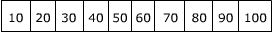# Percentiles and Quartiles

Percentiles divide a data set into 100 equal parts. A percentile is simply a measure that tells us what percent of the total frequency of a data set was at or below that measure. For example, let's consider a student's percentile on the ACT. If on this test, a given test taker scored in the 60th percentile on the quantitative section, she scored at or better than 60% of the other test takers. Further, if a total of 500 students took the test, she scored at or better than (500)x(.60) = 300 students who took the test. This means that 200 students scored better than she did.

As the name suggests, quartiles break the data set into 4 equal parts. The first quartile, Q1, is the 25th percentile. The second quartile, Q2, is the 50th percentile. The third quartile, Q3, is the 75th percentile. It's important to note that the median is both the 50th percentile and the second quartile, Q2.

Let's look at the following 10 data points.The range is 100 - 10 = 90. The minimum value is 10, and the maximum value is 100. Because there are ten values (an even number of values), the median is halfway between the 5th and 6th data values, which gives us 55 as the median, or Q2.

What if we were asked for the 25th percentile? We know that the 25th percentile is the first quartile (Q1). It is easy to find the first quartile.The first quartile (Q1) is just the "median" of all the values to the left of the true median. We can see that 30 is the middle number of the numbers to the left of the true median, so 30 is the 25th percentile and the first quartile (Q1).

What if we were asked for the 75th percentile? We know that the 75th percentile is the third quartile (Q3). The third quartile (Q3) is similarly the "median" of the values to the right of the true median. We can see that 80 is the middle number of the numbers to the right of the true median, so 80 is the 75th percentile and the third quartile (Q3).

We can summarize all this as follows:The five values that are bolded are called the 5-number summary, which will be used to create a boxplot, which is another graphical display of the data.
 Related Links: Math Probability and Statistics Boxplot (Box and Whiskers Diagram) Measures of Spread: Range, Standard Deviation, and Variance

To link to this Percentiles and Quartiles page, copy the following code to your site: